# Catch up on the latest AI articles# You Can Attack A Neural Network Just By Changing The Data Order!

3 main points
✔️ Attack technique by changing data batch order
✔️ Exploits the stochastic nature of the learning process
✔️ Demonstrated degradation of model performance, resetting of learning progress, and backdoor attacks

written by Ilia ShumailovZakhar ShumaylovDmitry KazhdanYiren ZhaoNicolas PapernotMurat A. ErdogduRoss Anderson
(Submitted on 19 Apr 2021 (v1), last revised 5 Jun 2021 (this version, v2))

Subjects: Machine Learning (cs.LG); Artificial Intelligence (cs.AI); Cryptography and Security (cs.CR); Computer Vision and Pattern Recognition (cs.CV)code：The images used in this article are from the paper, the introductory slides, or were created based on them.

## first of all

Machine learning models can be attacked by contamination of their training data, such as degradation of trained model performance and introduction of backdoors.

However, such an adversarial attack would require the attacker to be able to manipulate the data used for learning, which may not be realistic.

The paper presented in this article showed that it is possible to influence the behavior of the model by simply changing the batches and the order of the data during training, rather than changing the data for training as in existing attacks.

### Overall pipeline

The proposed method, Batch Reordering, Reshuffling and Replacing (BRRR) attack, is based on manipulating the order of batches during training and the order of data in a batch.

This attack is a black box attack where the attacker does not have access to the model under attack and follows the following pipeline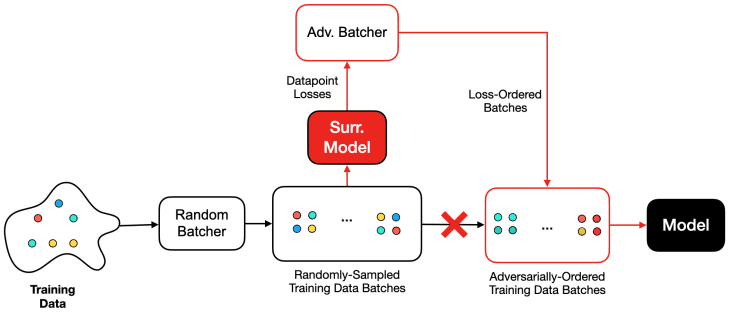Instead of not having access to the model under attack, the attacker trains the surrogate model in parallel and based on its output, reorders the batch or its data during training or replaces it with another data in the dataset.

At this time, no processing such as adding noise to the data is performed.

### Background of the BRRR attack

It can be said that the proposed attack technique exploits the probabilistic nature of current deep neural networks.

First, assume that the model under attack is a deep neural network with parameters $\theta$, a training dataset $X=\{X_i\}$, and a loss function $L(\theta)$. If the loss corresponding to the $i$th data point is $L_i(\theta)=L(X_i,\theta)$, then the loss average in the $k$th batch (size $B$) is $hat{L}_{k+1}(\theta)=frac{1}{B}\sum^{kB+B}_{i =kB+1}L_i(\theta)$. Now, if the number of samples in the entire training is $N \cdot B$, the loss we want to optimize is $hat{L}(\theta)=frac{1}{N}\sum^N_{i=1}\hat{L}_i(\theta)$.

In this case, for the learning rate $\eta$, the weight update in the SGD algorithm is expressed by the following equation

• $\theta_{k+1}=\theta_k+\eta \theta \theta_k$
• $\Delta \theta_k=-\nabla_\theta\hat{L}_k(\theta_k)$

In this case, the parameters after $N$ times SGD step are as follows.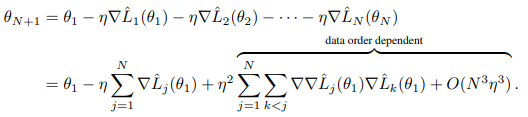As shown in this equation, the final parameter $\theta_{N+1}$ contains a term that depends on the order of the batches during training, which is indicated by data order dependence. The proposed method attacks this order-dependent term by manipulating it, e.g., degrading the performance of the final model.

This is an exploitation of a property of the learning algorithm that assumes that the batch sampling procedure is unbiased.

While the gradient of a mini-batch can be considered to approximate the true gradient if the sampling procedure is unbiased, the proposed method exploits and attacks this assumption by manipulating the data batch order by artifice.

### Classification of BRRR attacks

BRRR attacks are divided into three types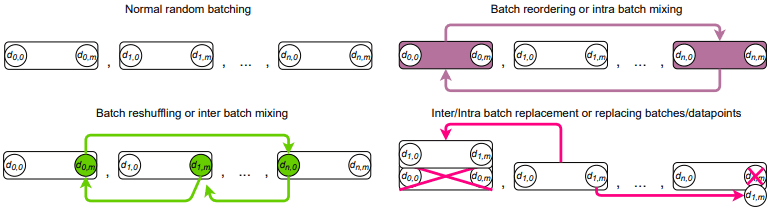• Batch reshuffling: Changes the order of data points in a batch (the number of occurrences of a data point is not changed).
• Batch reordering: Changes the order of the batch (the data points in the batch are not changed in content or order).
• Batch replacement: replaces a batch data point (the number of occurrences of the data point may be changed).

Here, the policy of changing the batch or data point order is classified in the following figure.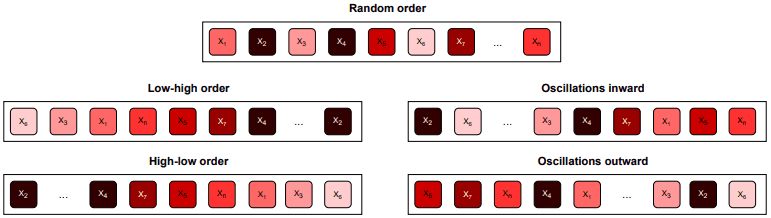• Low-High: Sort the loss from the lowest to the highest.
• High-Low: Sort the loss from high to low.
• Oscillation inwards: alternating order of the highest and lowest losses.
• Oscillations outward: Alternating order of the smallest loss above the median and the largest loss below the median.

These attacks are executed according to the following pseudo-algorithm.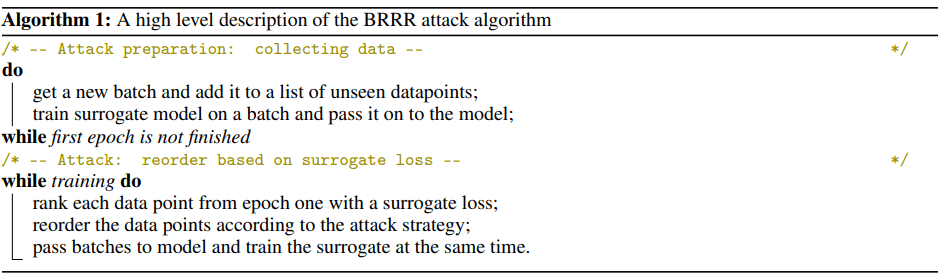For a more detailed pseudo-algorithm, please see Algorithm 2 in the original paper.

### Batch-Order Poisoning (BOP) and Backdooring (BOB) attacks

Poisoning backdoor attacks on machine learning models are usually done by adding a hostile data point $\hat{X}$ to the training time data set $X$ or by changing the data point to $X+\delta$.

Batch reordering attacks can also be applied to these poisoning backdoor attacks.

Specifically, the gradient corresponding to a hostile data point $\hat{X}_k$ is approximated by a data point $X_i$ with a similar gradient to it ($\nabla_\theta \hat{L}(\hat{X}_k,\theta_k) \approx \nabla_\theta \hat{L}(X_i,\theta_k)$).

In this case, the parameter update rule is as follows.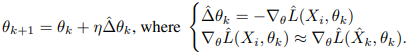This attack technique is a poisoning backdoor attack that can be carried out without modifying the original data set, making it a powerful attack that can be very difficult to detect and defend against.

## experimental results

In our experiments, we use the CIFAR-10, CIFAR-100, and AGNews datasets. In CIFAR-10 and CIFAR-100, we use ResNet-18 and ResNet-50 as source models (attackers), and LeNet-5 and MobileNet as surrogate models of attackers. AGNews uses three fully coupled layers as source models and one fully coupled layer as a surrogate model.

In general, the attacker's surrogate model is set to a lower performance model compared to the source model.

### Integrity attacks

The best performance of each source model with Batch reshuffling and Batch reordering is shown in the following table.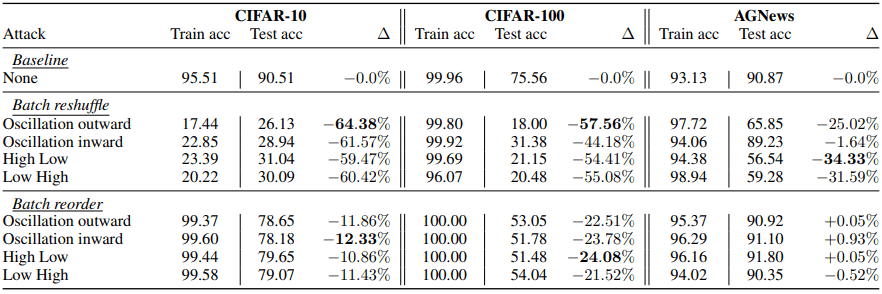(See the original paper Table 4 for more detailed results.)

In general, we find that the Batch reordering attack works well on the computer vision task, while the Batch reshuffling attack works well on any task. In addition, the best performance of each source model under the Batch reshuffling attack is at the first epoch, when the attacker has not been able to learn the dataset, and the performance drops to below random prediction for most epochs after that.

An example learning curve for the Batch reshuffling attack on ResNet18 is shown below.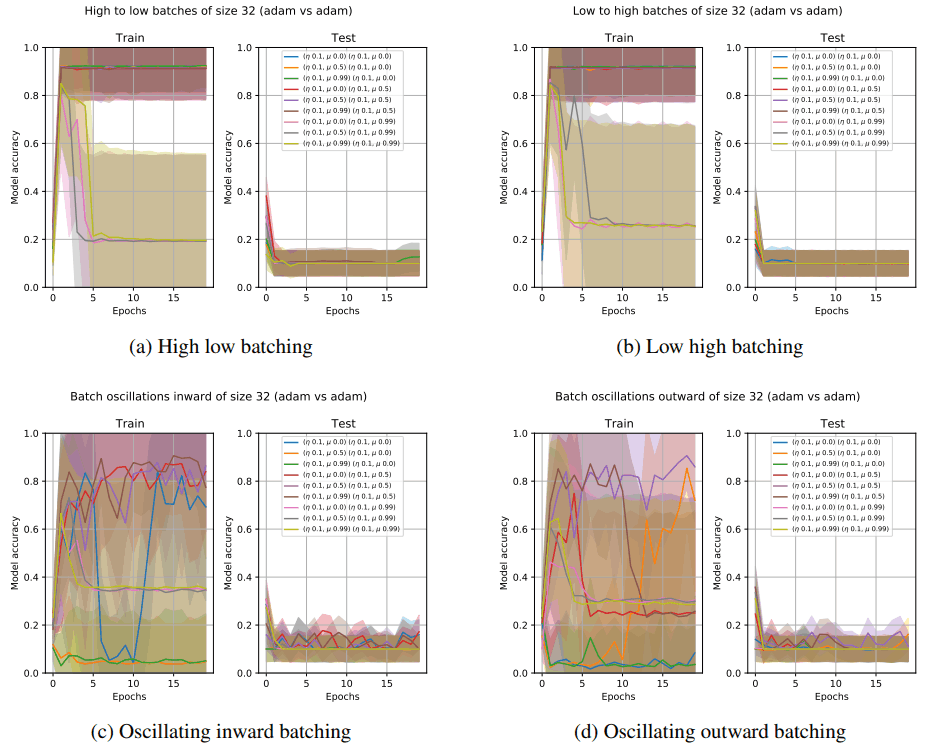In general, we found that changing the data point order or batch order can degrade the performance of the model and reset the training results.

### Availability attacks

Next, we consider availability attacks. In this section, we experiment to see if we can delay the training of the model when we perform an attack at a particular epoch.

The result of this is shown in the following figure.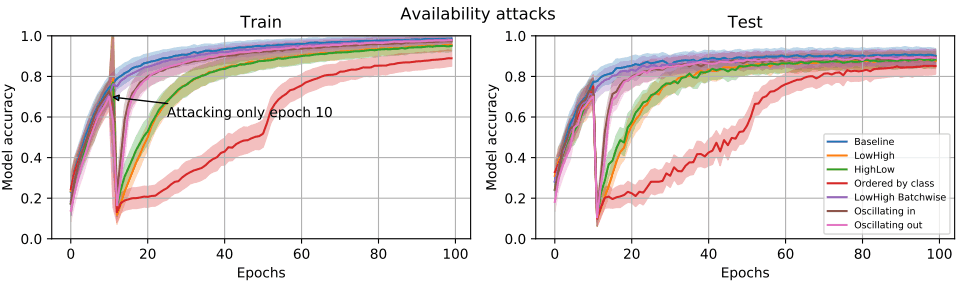In this figure, the reordering attack is performed only in 10 epochs. We have seen that a successful attack can be a very large threat, as it significantly resets the learning state and requires many epochs to return to its original performance.

### backdoor attack

Finally, we experiment with a backdoor attack by changing the batch order.

In this section, we perform the BOB attack described above on the image containing the trigger shown in the following figure.

The results are as follows (see the original paper for details of the setup).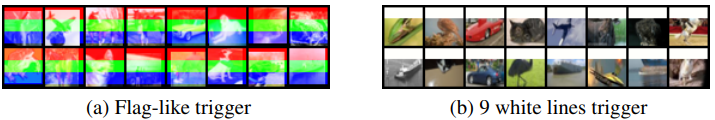In general, it was shown that a backdoor can be introduced without changing the original data by simply inserting a small amount of reordered batches, although the performance varies depending on the type of trigger and whether it is a black box or not.

## summary

We introduced a paper that proposed a new attack method that only requires changing the order of batches or data points, unlike existing attack methods that require changing the training data. Surprisingly, they even show that backdoor attacks can be performed by changing the order of the data points, which is a significant departure from existing attack methods and represents a new threat.

If you have any suggestions for improvement of the content of the article,